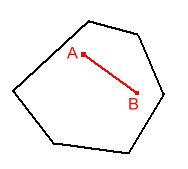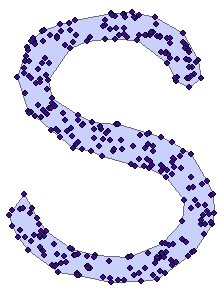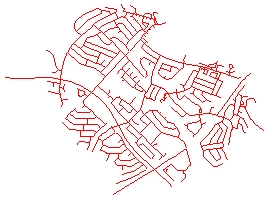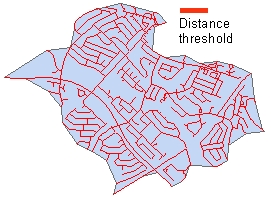## Create Concave Hull

Running programmatically

The Concave Hull function creates a polygon that represents the area occupied by a set of data points.

• The resulting polygon might be concave or convex

ConvexNon Convex (Concave)• A Concave hull describes better the shape of the point cloud than the convex hull

Convex HulConcave Hull• Many solutions are possible for the same input data. The result depends on the user defined distance threshold. The larger the threshold, the closer the resulting polygon will be to the Convex Hull.

Source DataConvex HullConcave Hull 1Concave Hull 2### Inputs:

• A feature layer (Point, Multipoint, Polyline, Polygon)
• Distance threshold - in the units of the spatial reference of the input dataset

### Outputs:

• New polygon layer.

### Running Programmatically

(Go to TOP)

Parameters

Expression Explanation
Function Name CreateConcaveHull
<input dataset> A String representing the input layer. Must be of Polygon type.
<output dataset> A String - the full name of the output layer.
<Distance Treshold> A Double representing the threshold for creating a concave hull - in the units of the spatial reference of the input dataset.

### Running the function

ETGWPath used in the table below is the  full path to ETGWRun.exe (E.G. "C:\Program Files\ETSpatial Techniques\ETGeo Wizards\ETGWRun.exe")

Language Syntax
Python subprocess.call([ETGWPath, "CreateConcaveHull", "input dataset", "output dataset", "Distance Treshold"])
.NET using ETGWRun.exe StartInfo.FileName = ETGWPath
StartInfo.Arguments = "CreateConcaveHull" "input dataset" "output dataset" "Distance Treshold"
.NET using ETGWOutX.dll CreateConcaveHull(input dataset, output dataset, Distance Treshold)
ArcPy arcpy.CreateConcaveHull(input dataset, output dataset, "Distance Treshold")

Notes:

• <> - required parameter

• {} - optional parameter

• See examples for Python , .NET or ArcPy

• The argument separator for StartInfo.Arguments is space. If a string might contain a space, you need to double quote it.

(Go to TOP)